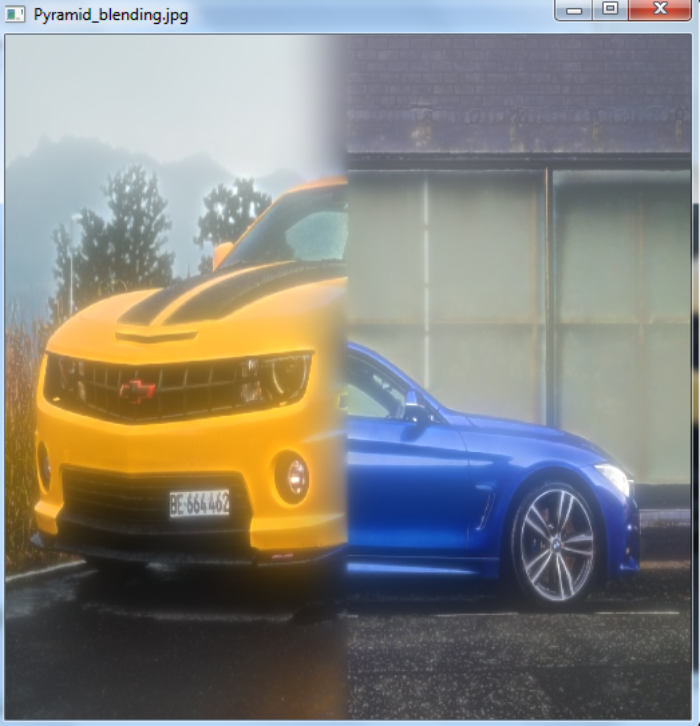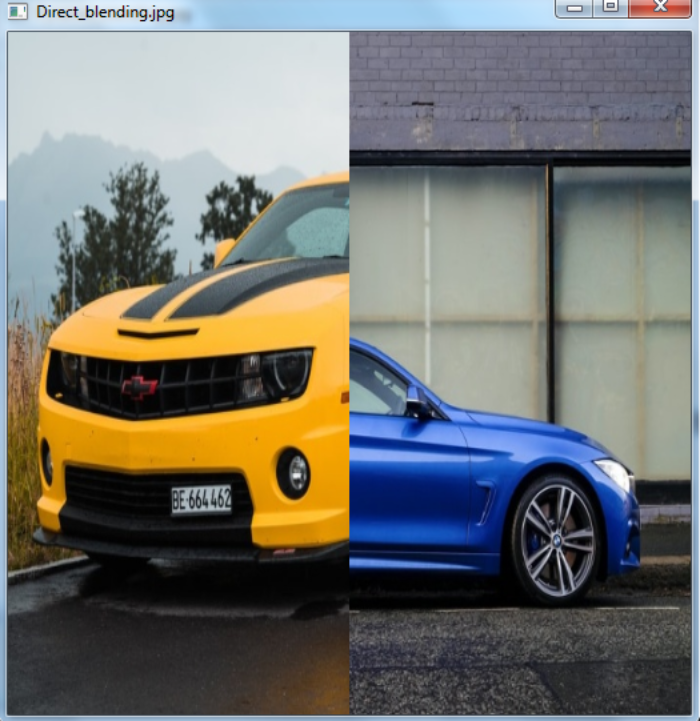# How to blend images using image pyramids in OpenCV Python?

We can blend the images using the Gaussian and Laplacian image pyramids. The Gaussian pyramid is a type of image pyramid. To create a Gaussian pyramid, OpenCV provides us two functions cv2.pyrDown() and cv2.pyrUp(). We can form the Laplacian Pyramids from the Gaussian pyramids. In Laplacian pyramid images look like edge images only. A level in the Laplacian Pyramid is formed by the difference between that level in the Gaussian Pyramid and the expanded version of its upper level in the Gaussian Pyramid.

## Steps

To blend images using image pyramids, we could follow the steps given below −

• Import the required libraries OpenCV and NumPy. Make sure you have already installed them.

• Read two input images A and B using cv2.imread() method. Specify the full image path. Resize the images to a size (512,512).

• Generate the Gaussian pyramids for both input images A and B.

• Using the Gaussian pyramids, generate the Laplacian pyramids for both input images A and B.

• Add the left half of the first image and right half of the second image in each level of Laplacian pyramids.

• Blend and reconstruct the image. Display the blended image

Let's have a look at some examples for more clear understanding.

## Input Images

We will use the following images as the input files in the example below.## Example

In this example, we blend two input images using the image pyramid.

import cv2
import numpy as np,sys
A = cv2.resize(A, (512, 512))
B = cv2.resize(B, (512, 512))

# generate Gaussian pyramid for A
G = A.copy()
gpA = [G]
for i in range(6):
G = cv2.pyrDown(G)
gpA.append(G)

# generate Gaussian pyramid for B
G = B.copy()
gpB = [G]
for i in range(6):
G = cv2.pyrDown(G)
gpB.append(G)

# generate Laplacian Pyramid for A
lpA = [gpA]
for i in range(5,0,-1):
GE = cv2.pyrUp(gpA[i])
L = cv2.subtract(gpA[i-1],GE)
lpA.append(L)

# generate Laplacian Pyramid for B
lpB = [gpB]
for i in range(5,0,-1):
GE = cv2.pyrUp(gpB[i])
L = cv2.subtract(gpB[i-1],GE)
lpB.append(L)

# Now add left and right halves of images in each level
LS = []
for la,lb in zip(lpA,lpB):
rows,cols,dpt = la.shape
ls = np.hstack((la[:,0:cols//2], lb[:,cols//2:]))
LS.append(ls)

# now reconstruct
ls_ = LS
for i in range(1,6):
ls_ = cv2.pyrUp(ls_)

# image with direct connecting each half
real = np.hstack((A[:,:cols//2],B[:,cols//2:]))
cv2.imshow('Pyramid_blending.jpg',ls_)
cv2.waitKey(0)
cv2.imshow('Direct_blending.jpg',real)
cv2.waitKey(0)
cv2.destroyAllWindows()


## Output

The above program, when executed, will produce the following two output windows −The first window shows image blending using the image pyramid and the second shows the direct image blending. Notice the difference between the above two images.

Updated on: 05-Dec-2022

608 Views